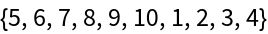Function Repository Resource:

RotateLeftUntil

Cycle the elements of a list to the left until a criterion is satisfied

Contributed by: Sander Huisman
 ResourceFunction["RotateLeftUntil"][expr,crit] cycles the elements in expr positions to the left until the first element satisfies crit. ResourceFunction["RotateLeftUntil"][expr,crit,n] cycles the elements in expr positions until crit has been satisfied n times. ResourceFunction["RotateLeftUntil"][expr,crit,n,m] cycles the elements in expr positions until crit has been satisfied n times with at most m iterations.

Details and Options

ResourceFunction["RotateLeftUntil"] is generally used to bring a certain element to the front.
The default value of n is 1.
When n is 0, the original expr is returned.
m is by default set to n×Length[expr].
\$Failed is returned if n fulfillments cannot be found in m iterations.

Examples

Basic Examples

Put the element "e" in front:

 In:=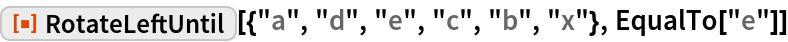Out=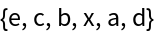Rotate until the fourth prime is in front:

 In:=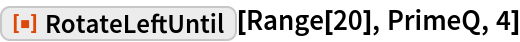Out=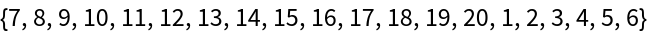Scope

Rotate until the first 5 is in front:

 In:=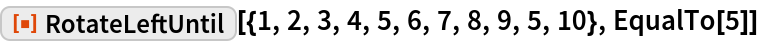Out=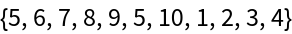Rotate until a value greater than or equal to 4 has been seen three times:

 In:=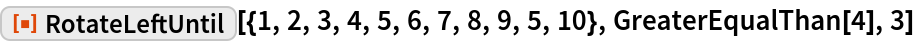Out=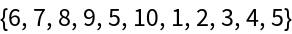Find the seventh occurrence of a value between 5 and 7, with a maximum of one hundred iterations:

 In:=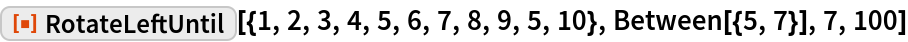Out=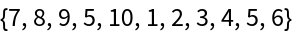Applications

Rotate the letters until a capital letter is at the front:

 In:=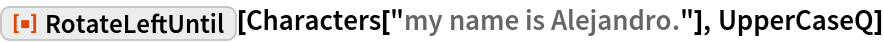Out=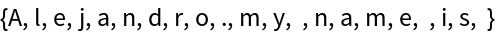Properties and Relations

RotateLeftUntil is related to RotateLeft and Position:

 In:=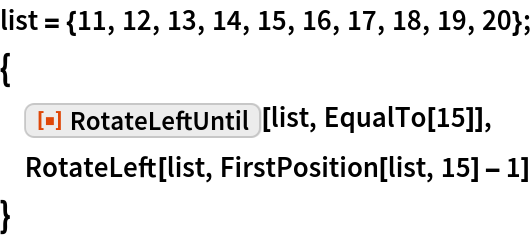Out=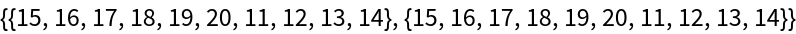Possible Issues

If the condition is not fulfilled within a cycle, \$Failed is returned:

 In:=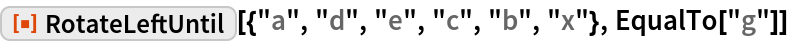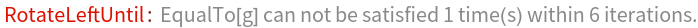Out=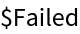For crit that change, more than n cycles might be needed:

 In:=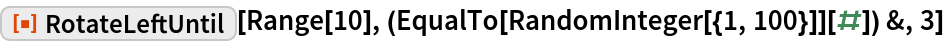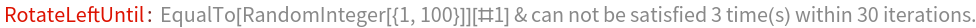Out=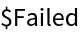Increase the maximum number of iterations:

 In:=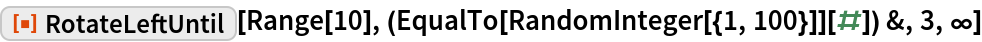Out=